## 6. The complex half-plane model for the hyperbolic plane

#### First, review complex numbers! From now on we use the properties of complex numbers!

The model that we start with is called the the upper half-plane model and it is defined to be:This simply says that we consider all the complex numbers that have the imaginary part strictly positive, i.e. if, then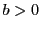.

The notion of angle inis the one inherited from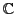, so the angle between two curves is the angle between their tangent lines.

Before we prove anything, let's do some warm-up exercises.

### Exercises

Exercise 1   Express the equations of the Euclidean line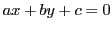and the Euclidean circlein terms of the complex coordinates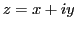.

Exercise 2   Let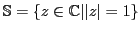be the unit circle in the complex plane. Letbe a Euclidean circle in the complex plane, with Euclidean centerand Euclidean radius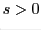. Show thatis perpendicular to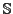if and only if.

You may wonder how this hyperbolic world looks like in this model. A good way to imagine it is to see how lines look like in this new world.

### Hyperbolic Lines

There are two seemingly different types of hyperbolic lines, both defined in terms of Euclidean objects in. One is the intersection of the half-plane with a Euclidean line in the complex plane perpendicular to the real axis. The other is the intersection ofwith a Euclidean circle centered on the real axis. Here is a picture of hyperbolic lines: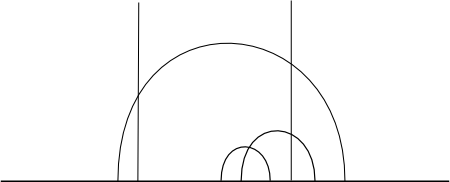Since the only difference between non-Euclidean and Euclidean geometry is the fifth postulate, the first four should hold. Using just our definition above, we should see that those four postulates hold. The proof is very tedious, so we will only show the first one: there should exist one and only one hyperbolic line passing through any pair of distinct points. Let's see how this follows from our definition:

Proposition 1   For each pair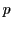and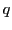of distinct points in, there exists a unique hyperbolic line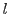passing throughand.

PROOF.

This is a typical existence and uniqueness problem. We will first show that such a line exists, and then that it is unique. We divide the proof into two parts: existence and uniqueness.

Existence

There are two cases to consider. Suppose first that. Then the Euclidean line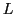given by the equation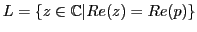is perpendicular to the real axis and passes through bothand. So the desired line is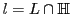.

Suppose now that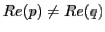. As the Euclidean line throughandis no longer perpendicular to the real line, we need to construct a Euclidean circle centered on the real axis and passing through the two points.

Denote withthe Euclidean line segment joining the two points, and let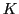be the perpendicular bisector of. Then (and this you should know from Euclidean geometry), every Euclidean circle that passes throughandhas its center on. Asandhave non-equal real parts, the Euclidean lineis not parallel to the real axis, sointersects the real axis at a unique point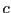.

Letbe the Euclidean circle, centered at this point, with radius(the two are equal, since the circle passes through both points) (see figure). So we have the line,.Uniqueness

The uniqueness of the line follows from the uniqueness of the Euclidean lines and the Euclidean circle. The proof of the first postulate is complete.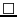Note that since we have chosen the underlying space for this model of the hyperbolic plane to be contained in the complex plane, we can use whatever facts about Euclidean lines and Euclidean circles we already know to analyse the behaviour of hyperbolic lines.

For example, ifis the hyperbolic line inpassing throughand, we can expressexplicitly in terms ofand. Like we saw above, if the two have the same real parts, then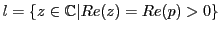. For the second case, do the following exercise:

Exercise 3   Letandbe distinct points inwith non-equal real parts, and letbe the Euclidean circle centered onand passing throughand. Express the Euclidean centerand the Euclidean radius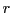ofin terms of,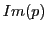,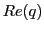,.

Hyperbolic geometry behaves very differently from Euclidean geometry in, even though it is expressed in terms of the latter.

Definition 1   Two hyperbolic lines inare parallel if they are disjoint.

Now we can see a first difference: in Euclidean geometry, parallel lines are equidistant, and so there is only one parallel through a given point to a given line, but in hyperbolic geometry things are completely the opposite - there are infinitely many distinct parallel lines through a given point to a given line.

In the figure below you can see how parallel lines look in our model of the hyperbolic plane: lines 1, 3, 4, 5, 6 are parallel, but 2, 3, 6, 7, 8 are not. Try to find all the pairs of parallel lines.You may wonder how polygons, circles and other figures look in hyperbolic geometry. The half-space model is not very good to visualise these objects, it is mostly used for computation or proving different properties (even if there are different models, the properties are the same for all, since they all describe the same concept, hyperbolic geometry). This is the reason why the next model, the Poincaré disk, is used for visualisation.

 INDEX NEXT